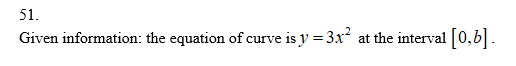# In Exercises 51–54, use a definite integral to find the area of the region between the given curve and the x-axis on the interval [0, b] .51. y = 3x252. y = 2x53. y = pai x254. y = x /2 + 1

Question
28 views

In Exercises 51–54, use a definite integral to find the area of the region between the given curve and the x-axis on the interval [0, b] .

51. y = 3x2

52. y = 2x

53. y = pai x2

54. y = x /2 + 1

check_circle

Step 1

Hey, since there are multiple questions posted, we will answer first questions. If you want any specific question to be answered, then please submit that question only or specify the question number in your message

Step 2Step 3

Write the equation of the...

### Want to see the full answer?

See Solution

#### Want to see this answer and more?

Solutions are written by subject experts who are available 24/7. Questions are typically answered within 1 hour.*

See Solution
*Response times may vary by subject and question.
Tagged in

### Calculus